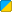# math.Approach

 Function Syntax math.Approach( Number Start, Number End, Number Incrementing ) Description: Increment the first number by the value of the last till it reaches the second number. Returns: Number Part of Library: Math Realm:BBCode Link: [b][url=http://wiki.garrysmod.com/?title=Math.Approach]Math.Approach [img]http://wiki.garrysmod.com/favicon.ico[/img][/url][/b]

## Examples

Description Demonstrates what effect the math.Approach function has on numbers```local num = 0 num = math.Approach(num, 20, 2) //Approach 20, incrementing by 2 --The variable num would now be 2, since it increased with 2. num = math.Approach(num, 0, 1) //Approach zero, incrementing by 1 --The variable num would now be 1, since it decreased with 1.``` N/A

Another way to put it

If you don't understand the above, what math.Approach does is when you call this function, there are three parameters needed. The first one is what value to modify. The second one is the value the first value will "approach". The final one is how much the value will increase. For example, if used in the Think portion of an entity, and use
```
self.A=math.Approach(self.A,self.B,25)
```

while self.A=0 and self.B=100 then A will automatically increase by 25 until it gets to the second parameter's value.